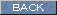| Japanese | English |

### REVERBERATION TIME OF BERLIN PHILHARMONIC HALL (Measurement of piano music 3)

Room reverberation is generally evaluated by the physical quantity "reverberation time." Reverberation time is defined as the amount of time required for the sound to decay 60 dB (one millionth) of the original level. Practically, reverberation time is calculated by a line fit to the first portion of the decay curve, instead of measuring the complete decay of 60 dB. It is common to use a line fit between -5 and -35dB, -5 and -25dB, or -5 and -15dB, and fitted line is extrapolated to 60dB. These values are specifically called as T30, T20 and EDT (early decay time). The decay curve is calculated from a room impulse response measured by using the impulsive sound (pistol or balloon), or MLS (maximum length sequence) or the TSP (time stretched pulse) signals. See also Audio measurement report 3 for more information about the reverberation time.

This time, I try to calculate the reverberation time of the hall by directly analyzing the music signal recorded in the hall. A piece from Karajan's piano concerto is analyzed. This is the Berlin philharmonic's recording of EDVARD GRIEG Piano Concerto in A minor, op.16.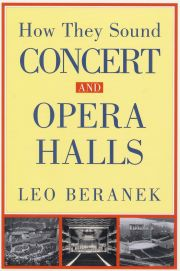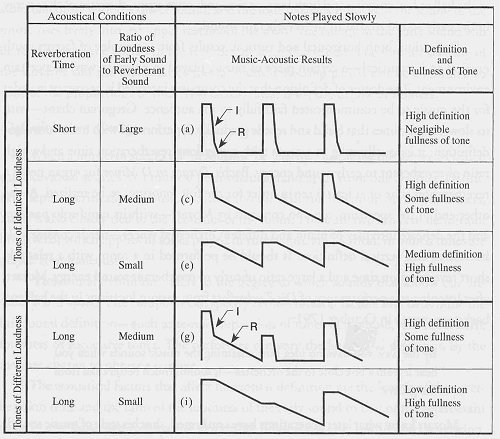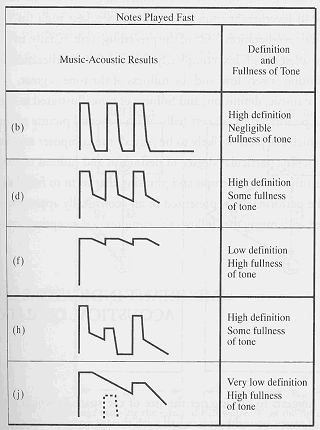This figure shows the time course of the sound level (Phi(0)) measured by the running ACF. The decay of the instrument (I) and the decay of the room sound (R) are also illustrated.From the figure, R (decay of room sound) takes 0.5 s to decay 10 dB (from -10 to -20 dB) , it means that it takes 3.0 s to decay 60 dB. Similarly, I (decay of instrument) takes 0.2 s to decay 10 dB, it means that it takes 1.2 s to decay 60 dB. Then, the difference of R and I, that is 1.8 s, might be the reverberation of the room.

Generally, the reverberation time is calculated for each octave band and is evaluated by the value for  500 Hz. In Beranek's How They Sound Concert and Opera Halls, pp604, measured reverberation times of Berlin philharmonic hall are listed. From the table below, averaged reverberation time is 1.85 s for the occupied conditions. Measured value by the running ACF seems acceptable.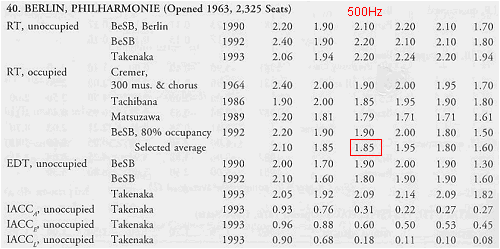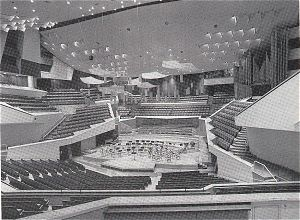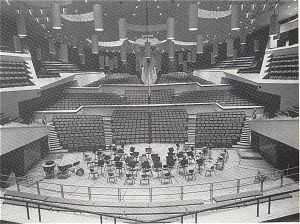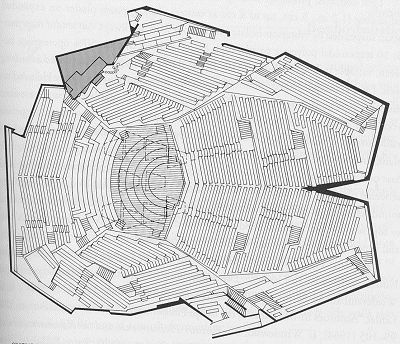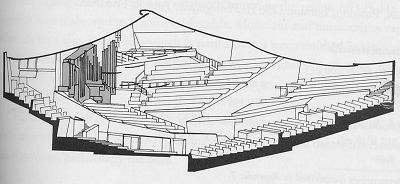The analyzing method introduced this time is how to find the reverberation time using running ACF measurement. Although this is the method that nobody has still performed, it seems clear that the high temporal resolution analysis clarifies all about room reverberation. You can get the same result. Download the wave file and try analysis.

Reference: How They Sound Concert and Opera Halls / Leo Beranek
(Published for the Acoustical Society of America through the American Institute of Physics)

April 2003 by Masatsugu Sakurai